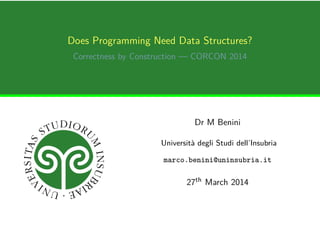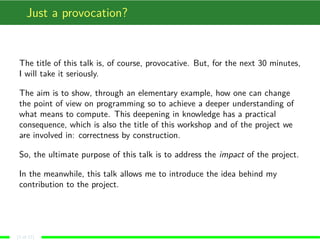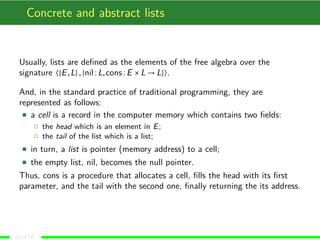Successfully reported this slideshow.

# CORCON2014: Does programming really need data structures?

0

Share×
1 of 17
1 of 17

# CORCON2014: Does programming really need data structures?

0

Share

This talk tries to suggest how computer programming can be conceptually simplified by using abstract mathematics, in particular categorical semantics, so to achieve the 'correctness by construction' paradigm paying no price in term of efficiency.
Also, it introduces an alternative point of view on what is a program and how to conceive data structures, namely as computable morphisms between models of a logical theory.

This talk tries to suggest how computer programming can be conceptually simplified by using abstract mathematics, in particular categorical semantics, so to achieve the 'correctness by construction' paradigm paying no price in term of efficiency.
Also, it introduces an alternative point of view on what is a program and how to conceive data structures, namely as computable morphisms between models of a logical theory.

## More Related Content

### Related Books

Free with a 14 day trial from Scribd

See all

### Related Audiobooks

Free with a 14 day trial from Scribd

See all

### CORCON2014: Does programming really need data structures?

1. 1. Does Programming Need Data Structures? Correctness by Construction — CORCON 2014 Dr M Benini Università degli Studi dell’Insubria marco.benini@uninsubria.it 27th March 2014
2. 2. Just a provocation? The title of this talk is, of course, provocative. But, for the next 30 minutes, I will take it seriously. The aim is to show, through an elementary example, how one can change the point of view on programming so to achieve a deeper understanding of what means to compute. This deepening in knowledge has a practical consequence, which is also the title of this workshop and of the project we are involved in: correctness by construction. So, the ultimate purpose of this talk is to address the impact of the project. In the meanwhile, this talk allows me to introduce the idea behind my contribution to the project. (2 of 17)
3. 3. Concrete and abstract lists Usually, lists are deﬁned as the elements of the free algebra over the signature 〈{E,L},{nil:L,cons:E ×L → L}〉. And, in the standard practice of traditional programming, they are represented as follows: a cell is a record in the computer memory which contains two ﬁelds: the head which is an element in E; the tail of the list which is a list; in turn, a list is pointer (memory address) to a cell; the empty list, nil, becomes the null pointer. Thus, cons is a procedure that allocates a cell, ﬁlls the head with its ﬁrst parameter, and the tail with the second one, ﬁnally returning the its address. (3 of 17)
4. 4. Concrete manipulation of a list As an example of program, we consider the concatenate function. Its speciﬁcation is: “Given the lists [x1,...,xn] and [y1,...,ym], concatenate has to return the list [x1,...,xn,y1,...,ym]”. Usually, it is implemented as concatenate(x,y) ≡ if x = nil then return y else q := x while q = nil do p := q q := q → tail p → tail := y return x (4 of 17)
5. 5. Correctness The previous algorithm is correct. In fact, when x = nil, it returns y, satisfying the speciﬁcation. When x = nil, x = [x1,...,xn]. So, at the end of the i-th iteration step, p = [xi ,...,xn] and q = [xi+1,...,xn], as it is immediate to prove by induction. Also, the cycle terminates after n iterations, and p = [xn]. But, in the concrete representation of x, p → tail must be nil and the assignment p → tail := y substitutes nil with y. So x = [x1,...,xn,y1,...,ym], as required. The proof sketched above uses in an essential way the concrete representation of the x list, because the algorithm uses “list surgery”. It is evident that the algorithm, and, thus, its correctness proof, is hardwired. (5 of 17)
6. 6. A functional derivation Dropping list surgery, we can use the abstract formalisation of lists directly: concatenate(x,y) ≡ if x = nil then return y else return cons (hdx) (concatenate(tlx,y)) where hd and tl return the head and the tail of its argument, respectively. Of course, this is a functional program, and it is justiﬁed by the following reasoning, which can be immediately converted into a formal correctness proof by induction on the structure of x: 1. we want that concatenate([x1,...,xn],[y1,...,ym]) = [x1,...,xn,y1,...,ym], 2. as before, if x = nil, the result is just y 3. when x = nil, concatenate([x1,...,xn],[y1,...,ym]) yields the same result as consx1 (concatenate([x2,...,xn],[y1,...,ym])); 4. in the line above, the recursive application decreases the length of the ﬁrst argument, so recursion terminates after n steps. (6 of 17)
7. 7. Recursion versus induction In the functional implementation of concatenate, we may interpret the recursive schema as the computational counterpart of an inductive schema. It is immediate to see that such an inductive schema becomes the skeleton of the correctness proof. So, the functional program “carries” with itself a proof of correctness, in some sense. Usually, the functional implementation of concatenate is regarded as ineﬃcient because it recursively constructs a number of intermediate lists before yielding the ﬁnal results. Often, this is said to be the inevitable eﬀect of dropping list surgery. (7 of 17)
8. 8. Abstracting over lists We formalised a list [x1,...,xm] as consx1 (consx2 (...(consxm nil)...)). We can use a slightly diﬀerent representation1: λn,c. c x1 (c x2 (...(c xm n)...)) . The key idea is to abstract over the structure of the data type, making it part of the representation of the datum. Alternatively, we can interpret this representation A as the abstract datum, and the concrete one, C can be obtained by passing the instances of the constructors A. For example, the standard formalisation is obtained by Anilcons. 1As far as I know, the general algorithm to derive such a representation is due to Böhm and Berarducci, and it can be traced back to Church (8 of 17)
9. 9. Interpreting abstract lists An abstract list can be thought of as representing a term in the ﬁrst-order logical language with the equality relation symbol, and the signature of the data type of lists. The λ-term standing for the abstract list realises the mapping from the logical term — the list, the body of the abstraction — into some model, which is speciﬁed when we apply to the λ-term the way to interpret the function symbols. If we ﬁx this point of view, we can write a “correct by construction” implementation of concatenate: concatenate ≡ λx,y,n,c. x (y nc) c . (9 of 17)
10. 10. Correctness by construction I concatenate ≡ λx,y,n,c. x (y nc) c . It is worth explaining the construction of this program: 1. it is a function, which takes two argument x and y; 2. it returns an abstract list, so a λ-term of the form λn,c. L, with L a logical term in the language of lists; 3. the y abstract list gets interpreted in the same model as the result of concatenate — and this is rendered by y nc; 4. the x abstract list gets interpreted in a model which has the same interpretation for cons, but it interprets nil as the ‘concrete’ y. We should remark that, in fact, this abstract implementation is, in essence, the very same algorithm we have shown in the beginning, deprived from the irrelevant details about the concrete data structure of lists. (10 of 17)
11. 11. Correctness by construction II concatenate ≡ λx,y,n,c. x (y nc) c . The above deﬁnition is a direct coding of the explanation. In turn, the explanation can be converted into a correctness proof by observing that the structure depicted in point (4) is a model for the theory of lists; there is a mapping that preserves the meaning between the standard term model and the model above; this mapping is just the function concatenate. The idea behind this proof is that the function concatenate, intended as a program, is nothing but a morphism between models of the same theory. A non-evident aspect of the explanation of concatenate is that concatenate correctly operates in any model for the theory of lists. (11 of 17)
12. 12. One program, many meanings For example, natural numbers, described as the structure generated by zero and successor, are a model for lists: cons ≡ λe,l. sucl and nil ≡ 0. And concatenate becomes just the usual addition. For example, interpreting cons as the Cartesian product and nil as the terminal object in a category with products, we get another model for lists. And concatenate becomes just the Cartesian product of two products. For example, interpreting cons as function application and nil as the identity function, we get another model for lists. And concatenate becomes function composition. (12 of 17)
13. 13. Interpretations and computing A hidden aspect of interpreting a data type in a model is that computational patterns can be rendered explicitly. For example, if we take lists of trees as our model for lists, and we deﬁne hd as the list containing the root elements, and tl as the list of their sons, the abstract structure of a single tree corresponds to the procedure that sequentially scans the tree breadth-ﬁrst. (13 of 17)
14. 14. Generalising Does it work only for lists? The theory behind the abstract representation for data types has been developed by Böhm and Berarducci, and it directly applies to all the data structures that can be formalised as free algebras of terms over a ﬁrst-order signature. This holds for most of the elementary structures which are used in the current practice of programming. In a similar way, co-inductive data structures can be modelled as well. For data structures which are not free (co-)algebras, there are still some open problems, but, to some extent, they can be modelled in the same spirit. That is, representing data as functions whose parameters describe the “structure” of the data type. Does it work in a “real” programming language? As far as the programming language supports the dynamic creation of functions, e.g., by providing abstraction, the technique can be immediately used. (14 of 17)
15. 15. A philosophical remark The title of this talk was “Does programming really need data structures?”. Now, we can say that the answer is not immediately positive: (YES) programming needs data, and data must be structured to be represented and manipulated by a formal entity like a program; (NO) programming does not need concrete data structures. In fact, a program relies only on the structural properties of a data type to perform its computation: as far as these properties are accessible, for example, as explicit parameters, we can do without data structures; (YES) when we conceive a program, we assume to work on data represented according to some structure. It is possible (and, I claim, convenient) to make this structure abstract, but a structure is still present, and it shapes the way the computation is performed; (NO) the abstract structure we pass to our representation of data is nothing but an “interpretation” of a (logical) theory into a model. In fact, we do not need to know how the model is represented, but only how to express the mapping from the canonical model to the intended “world” where the computation is assumed to take place. (15 of 17)
16. 16. Conclusions In the previous slide there is a hidden assumption: that the logical theory has a “canonical” model which can be transformed into a generic model via a suitable mapping. This is not true in general. So the presented point of view can be stretched only when considering logical theories having such a classifying model — which is the case for free algebras of terms, for example. In my recent research, I’ve shown a semantics for ﬁrst-order intuitionistic logical theories, based on a categorical setting, which has classifying models. So, every such a theory could, in principle, be regarded as a “data structure” in the sense of this talk. My contribution to the CORCON research project will be to investigate whether semantics like the above one can be eﬀectively used to model data structures in an programming environment. Also, the side message of this talk is to show how even the most elementary aspects of our project may have a non-trivial impact to the current practice of programming. It is just a question of taking the “right” point of view, after all. . . (16 of 17)
17. 17. The end Tramonto, Rodi — © Marco Benini (2012) (17 of 17)